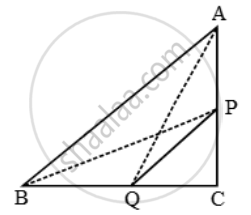Share

# P and Q are the mid-points of the sides CA and CB respectively of a ∆ABC, right angled at C. Prove that - CBSE Class 10 - Mathematics

#### Question

P and Q are the mid-points of the sides CA and CB respectively of a ∆ABC, right angled at C. Prove that:

(i) 4AQ^2 = 4AC^2 + BC^2

(ii) 4BP^2 = 4BC^2 + AC^2

(iii) (4AQ^2 + BP^2 ) = 5AB^2

#### Solution(i) Since ∆AQC is a right triangle right-angled at C.

∴ AQ^2 = AC^2 + QC^2

⇒ 4AQ^2 = 4AC^2 + 4QC^2

⇒ 4AQ^2 = 4AC^2 + (2QC)^2

⇒ 4AQ^2 = 4AC^2 + BC^2

(ii) Since ∆BPC is a right triangle right-angled at C.

∴ BP^2 = BC^2 + CP^2

⇒ 4BP^2 = 4BC^2 + 4CP^2

⇒ 4BP^2 = 4BC^2 + (2CP)^2

⇒ 4BP^2 = 4BC^2 + AC^2 [∵ AC = 2CP]

(iii) From (i) and (ii), we have

4AQ^2 = 4AC^2 + BC^2 and, 4BC^2 = 4BC^2 + AC^2

∴ 4AQ^2 + 4BP^2 = (4AC^2 + BC^2 ) + (4BC^2 + AC^2 )

⇒ 4(AQ^2 + BP^2 ) = 5 (AC^2 + BC^2 )

⇒ 4(AQ^2 + BP^2 ) = 5 AB^2

[In ∆ABC, we have AB2 = AC2 + BC2 ]

Is there an error in this question or solution?

#### Video TutorialsVIEW ALL 

Solution P and Q are the mid-points of the sides CA and CB respectively of a ∆ABC, right angled at C. Prove that Concept: Pythagoras Theorem.
S